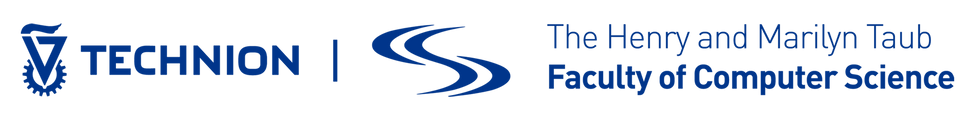top of page## Pre-FLoC workshops

• Third Joint International Workshop Linearity-TLLA

• Homotopy Type Theory/Univalent Foundations

• Meeting of the IFIP Working Group 1.6 (IFIP-WG1.6)

• Ninth International Workshop on Rewriting Techniques for Program Transformations and Evaluation (WPTE 2022)

• Women in Logic 2022

• Third Joint International Workshop Linearity (TLLA)

• Homotopy Type Theory/Univalent Foundations

• 11th International Workshop on Confluence (IWC 2022)

• International Workshop on Logical Frameworks and Meta-Languages: Theory and Practice (LFMTP)

• Computing with Terms and Graphs (TERMGRAPH)

• Parameterized Complexity and Computational Reasoning (PCCR)​​​​​​​​​​​​​​​​​​​​​​​​​​​​​​​​​​​​​​​​​​​​​​​​​​​​​

• Assumption-Based Nonmonotonic Reasoning

• Referring Expressions in Artificial Intelligence and Knowledge Representation Systems​​​​​​​​​​​​​​​​​​​​​​​​​​​​​​​​​​​​​​​​​​​​​​​​​​​​​​​​​​​​​​​​​​​​​​​​​​​​​​​​​​​​​​​​​​​​​​​​​​​​​​​​​​​​​​​​​​​​​​​​​​​​​​​​​​​​​​​​​​​​​​​​​​​​​​​​​​​​​​​​​​​​​​​​​​​​​​​​​​​​​​​​​​​​​​​​​​​​​​​​​​​​​​​​​​​​​​​​​​​​​​​​​​​​​​​​​​​​​​​​​​​​​​​​​​​​​​​​​​​​​​​​​​​​​​​​​​​​​​​​​​​​​​​​​​​​​​​​​​​​​​​​​​​​​​​​​​​​​​​​​​​​​​​​​​​​​​​​​​​​​​​​​​​​​​​​​​​​​​​​​​​​​​​​​​​​​​​​​​​​​​​​​​​​​​​​​​​​​​​​​​​​​​​​​​​​​​​​​​​​​​​​​​​​​​​​​​​​​​​​​​​​​​​​​​​​​​​​​​​​​​​​​​​​​​​​​​​​​​​​​​​​​​​​​​​​​​​​​​​​​​​​​​​​​​​​​​​​​​​​​​​​​​​​​​​​​​​​​​​​​​​​​​​​​​​​​​​​​​​​​​​​​​​​​​​​​​​​​​​​​​​​​​​​​​​​​​​​​​​​​​​​​​​​​​​​​​​​​​​​​​​​​​​​​​​​​​​​​​​​​​​​​​​​​​​​​​​​​​​​​​​​​​​​​​​​​​​​​​​​​​​​​​​​​​​​​​​​​​​​​​​​​​​​​​​​​​​​​​​​​​​​​​​​​​​​​​​​​​​​​​​​​​​​​​​​​​​​​​​​​​​​​​​​​​​​​​​​​​​​​​​​​​​​​​​​​​​​​​​​​​​​​​​​​​​​​​​​​​​​​​​​​​​​​​​​​​​​​​​​​​​​​​​​​​​​​​​​​​​​​​​​​​​​​​​​​​​​​​​​​​​​​​​​​​​​​​​​​​​​​​​​​​​​​​​​​​​​​​​​​​​​​​​​​​​​​​​​​​​​​​​​​​​​​​​​​​​​​​​​​​​​​​​​​​​​​​​​​​​​​​​​​​​​​​​​​​​​​​​​​​​​​​​​​​​​​​​​​​​​​​​​​​​​​​​​​​​​​​​​​​​​​​​​​​​​​​​​​​​​​​​​​​​​​​​​​​​​​​​​​​​​​​​​​​​​​​​​​​​​​​​​​​​​​​​​​​​​​​​​​​​​​​​​​​​​​​​​​​​​​​​​​​​​​​​​​​​​​​​​​​​​​​​​​​​​​​​​​​​​​​​​​​​​​​​​​​​​​​​​​​​​​​​​​​​​​​​​​​​​​​​​​​​​​​​​​​​​​​​​​​​​​​​​​​​​​​​​​​​​​​​​​​​​​​​​​​​​​​​​​​​​​​​​​​​​​​​​​​​​​​​​​​​​​​​​​​​​​​​​​​​​​​​​​​​​​​​​​​​​​​​​​​​​​​​​​​​​​​​​​​​​​​​​​​​​​​​​​​​​​​​​​​​​​​​​​​​​​​​​​​​​​​​​​​​​​​​​​​​​​​​​​​​​​​​​​​​​​​​​​​​​​​​​​​​​​​​​​​​​​​​​​​​​​​​​​​​​​​​​​​​​​​​​​​​​​​​​​​​​​​​​​​​​​​​​​​​​​​​​​​​​​​​​​​​​​​​​​​​​​​​​​​​​​​​​​​​​​​​​​​​​​​​​​​​​​​​​​​​​​​​​​​​​​​​​​​​​​​​​​​​​​​​​​​​​​​​​​​​​​​​​​​​​​​​​​​​​​​​​​​​​​​​​​​​​​​​​​​​​​​​​​​​​​​​​​

• CAUSAL 2022

• Workshop on Epistemic Extensions of Logic Programming (EELP)

• Workshop on Answer Set Programming and Other Computing Paradigms (ASPOCP 2022)

• School on ​Logic Programming

• How and why to teach logic for CS students?

• Decidable Fragments of First-order Modal Logic (DECFOML)

• How and why to teach logic for CS students?

## Aug 1st

### CP

• Workshop on Constraint Modelling and Reformulation (ModRef 2022)

# FLoC 2022 Participating Workshops

## Post-FLoC workshops

• Workshop on Verification of Probabilistic Programs (VeriProP 2022)

• Seventh Workshop on Satisfiability Checking and Symbolic Computation (SC²)

• The 36th International Workshop on Unification (UNIF 2022)

• Workshop on Termination (WST 2022)

• Practical Aspects of Automated Reasoning (PAAR-2022)

• Satisfiability Modulo Theories Workshop

• The Coq Workshop 2022

• Workshop on Counting and Sampling

## Aug 12th

### CSF## Fully virtual workshops

Dynamic Logic: new trends and applications (4th edition of DaLi) (LICS) >>

Advances in Separation Logic (IJCAR) >>

registration for virtual events are handled by each workshop.

bottom of page